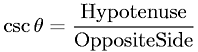Equations > Trigonometry > Trigonometric Definitions > Cosecant Definition for a Right Triangle

### Cosecant Definition for a Right TriangleLatex Code:

MathML Code:

 $\mathrm{csc}\theta =\frac\mathrm{Hypotenuse}\mathrm{Opposite}\mathrm{Side}$

MathType 5.0: